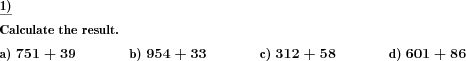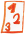Custom math worksheets at your fingertips# Details for problem "Add two numbers with specific no of places"

Quickname: 6327

## Summary

Addition of two numbers with controlled number of digits for each number.

## Example## Description

Two oositive natural numbers with no decimal places have to be added.

The number of digits for the first and second number can be chosen. It may be specified whether a carry over the leftmost place of the second number is allowed. This means for a two digit number a carry over to hundreds, to a three digit number a carry over to thousands etc.

The number of problems can be selected.

Download free worksheets for this math problem here. The worksheet contains the problems only, the solutions sheet includes the answers. Just click on the respective link.

•Worksheet 1Solution sheet with answers
•Worksheet 2Solution sheet with answers
•Worksheet 3Solution sheet with answers

If you can not see the solution sheets for download, they may be filtered out by an ad blocker that you may have installed. If this is the case, please allow ads for this page and reload the page. The solution sheets will then reappear.

• Do the sample worksheets do not really fit?
• Do you need more math worksheets, with a different level of difficulty?
• Would you like to combine different problems on a worksheet and adjust them to your needs?
• As a teacher, you can put together your own worksheets using the automatically generated math problems provided.
With a free initial credit, you can start creating your own math worksheets in a few minutes.

It does not cost anything to try! Register here, to create custom worksheets now!

## Customization options for this problem

Parameter
Possible values
Number of problems
1, 2, 3, 4, 5, 6, 7, 8, 9, 10
Digits number a
1, 2, 3, 4, 5, 6
Digits number b
1, 2, 3, 4, 5, 6
Tens carry >b digits
Never, May, Always

## Similar problems

Remark
Description
with multiples of powers of ten
Multiples of powers of ten added to natural numbers.
multiple summands in a defined number range, with or without decimals
Numbers with or without decimal places have to be added.
long addition of numbers with decimals
Columnar addition of multiple decimal fractions.
variant in the form of a matrix
Table of addition and subtraction problemsDeutsche Version dieser Aufgabe
These informational pages with samples describe math problems that can be combined on custom math worksheets with solutions for home and school use.
Deutsche Seiten
×